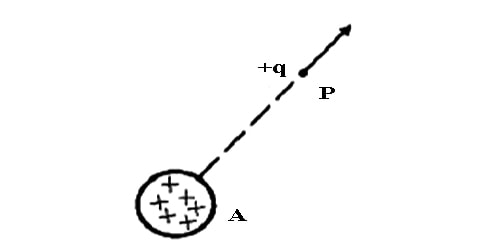# Electric Field

Suppose A is a positively charged body. Now if a charge +q is placed at point P, then due to the charge of A, the +q charge will gain a force. We say that at point P there is an electric field, the source of which a charged body A. That is if a charged body, in which the influence of the charged body exists is called the electric field of the charged body.Fig: Electric Field

Electric Intensity:

According to Coulomb’s law, it is found that the nearer the point P [Fig] to the charged body A, the more will be the strength of the electric field at that point. The strength of the electric field is called intensity. If at any point of an electric field a unit of positive charge is placed and the force that it acquires is called the electric intensity at that point. If the charge at point P acquires a force F, then the intensity of the electric field at that point P,

E = F/q

Electric intensity is a vector quantity and its direction is along the force acting on a unit positive charge placed in an electric field. The unit of electric charge is Newton/Coulomb (NC-1).